# GSEB Solutions Class 6 Maths Chapter 5 Understanding Elementary Shapes InText Questions

Gujarat Board GSEB Textbook Solutions Class 6 Maths Chapter 5 Understanding Elementary Shapes InText Questions and Answers.

## Gujarat Board Textbook Solutions Class 6 Maths Chapter 5 Understanding Elementary Shapes InText Questions

Try These (Page 88)

Question 1.
Take any post card. Use the above technique to measure its two adjacent sides.
Solution:
Do it yourself.

Question 2.
Select any three objects having a flat top. Measure all sides of the top using a divider and a ruler
Solution:
Do it yourself.Try These (Page 91)

Question 1.
What is the angle name for half a revolution?
Solution:
2 straight angles = 1 revolution
$$\frac { 1 }{ 2 }$$[2 straight angles] = $$\frac { 1 }{ 2 }$$[1 revolution]
or A straight angle = $$\frac { 1 }{ 2 }$$ revolution
Thus, half a revolution means a straight angle.

Question 2.
What is the angle name for one-fourth revolution?
Solution:
4 right angles = 1 revolution
A right angle = $$\frac { 1 }{ 4 }$$ revolution
Thus, one-fourth revolution means a right angle.Question 3.
Draw five other situations of one-fourth, half and three-fourth revolution on a clock
Solution:
(i) One-fourth revolution [i.e. a turn of a right angle]
For one-fourth revolution, the clock hand moves from:
(a) 12 to 3
(b) 3 to 6
(c) 1 to 4
(d) 9 to 12
(e) 6 to 9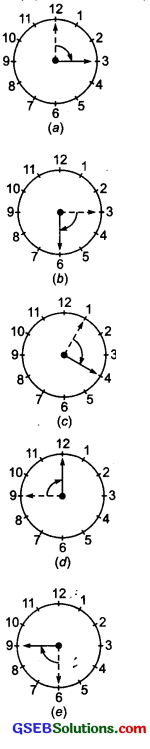(ii) Half-revolution [i.e. a turn of a straight angle]
For a half-revolution, the clock hand moves from:
(a) 12 to 6
(b) 3 to 9
(c) 1 to 7
(d) 2 to 8
(e) 4 to 10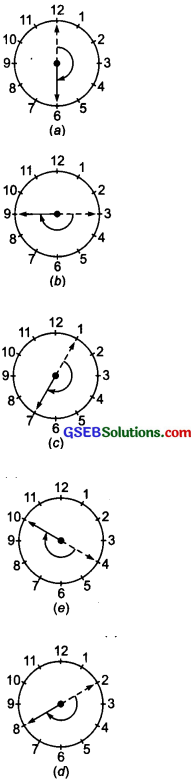(iii) Three-fourth revolution [i.e. a turn of 3 right angles]
Note: There is no special name for three-fourth of a revolution.
For a three-fourth revolution, the clock-hand moves from:
(a) 12 to 9
(b) 1 to 10
(c) 3 to 12
(d) 6 to 3
(e) 9 to 6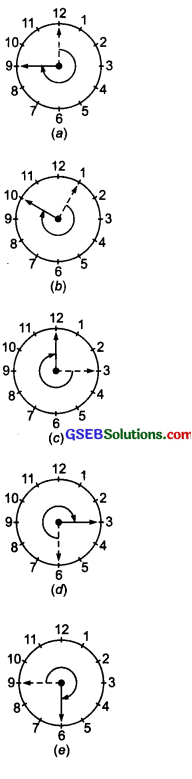Try These (Page 93)

Question 1.
The hour hand of a clock moves from 12 to 5. Is the revolution of the hour hand more than l right angle?
Solution:
Yes, the revolution of the hour hand from 12 to 5 is more than 1 right angle.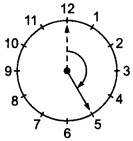Question 2.
What does the angle made by the hour hand of the clock look like when it moves from 5 to 7? Is the angle moved more than l right angle?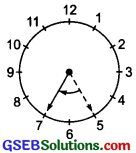Solution:
No, in this case the angle is less than a right angle.

Question 3.
Draw the following and check the angle with your RA tester.
(a) going from 12 to 2
(b) from 6 to 7
(c) from 4 to 8
(d) from 2 to 5
Solution:
(a) Going from 12 to 2: The angle formed by the hour hand in going from 12 to 2 is shown in the figure alongside. Checking this angle by RA [right angle] tester, we find that it is less than a right angle.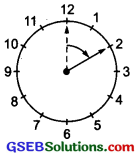(b) Going from 6 to 7: Checking the angle formed by RA tester, we find that it is less than a right angle.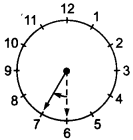(c) Going from 4 to 8: Checking the angle so formed by RA tester, we find that it is greater than a right angle.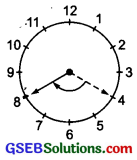(d) Going from 2 to 5: Checking the angle so formed by RA tester, we find that it is equal to a right angle.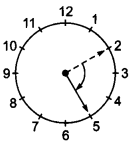Question 4.
Take five different shapes with corners. Name the corners. Examine them with your tester and tabulate your results for each case: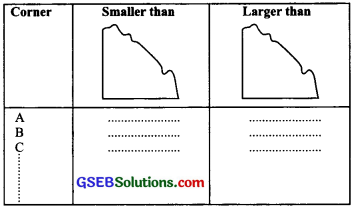Solution:
It is an activity. Do it yourself.

Try These (Page 94)

Question 1.
Look around you and identify edges meeting at corners to produce angles. List ten such situations.
Solution:
Do it yourself.

Question 2.
List ten situations where the angles made are acute.
Solution:
Do it yourself.Question 3.
List ten situations where the angles made are right angles.
Solution:
Do it yourself.

Question 4.
Find five situations where obtuse angles are made.
Solution:
Do it yourself.

Question 5.
List five other situations where reflex angles may be seen.
Solution:
Do it yourself.